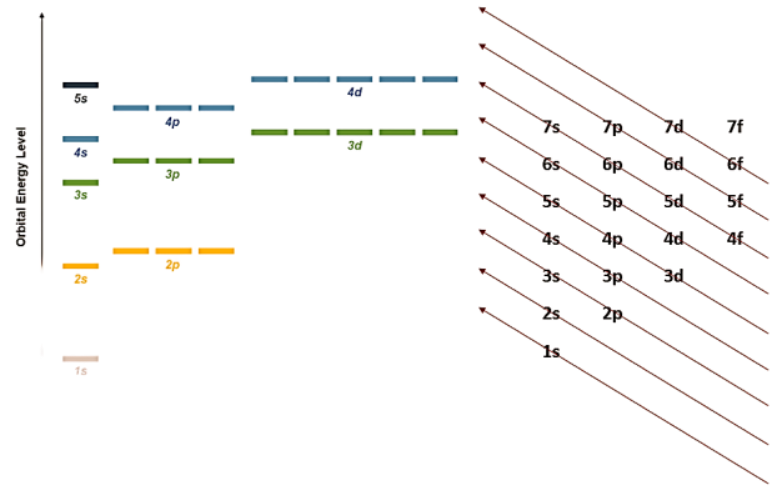# Aufbau Principle

The concept of electronic configuration provides several attributes of an atom, such as the shape and energy of its orbitals that are responsible for characteristic properties of each element. It also provides an insight towards how chemical bonds are formed between atoms. This notation presents the energy levels, orbitals and the number of the electron in an atom or molecule.

The word ‘Aufbau’ is derived from the German word ‘ Aufbauprinzip’ that can be roughly translated to ‘build up’ or ‘assemble’. This order of assembly can be derived using (n+l) rule, also called Madelung rule where ‘n’ stands for principal quantum number and ‘l’ stands for the angular quantum number. The sum of these numbers determines the energy level of the orbital in question. Hence, lower (n+l) values depict lower orbital energy. In cases where two orbitals share same (n+l) value, then orbital with lower ‘n’ value is associated with lower energy level (Singh, 2011).

The Aufbau principle serves as a starting point to determine the electronic configuration of an atom or ion. It states that the electrons must occupy the lowest energy orbital first, i.e. electrons will first fill the 1s orbital before occupying 2s orbital in its ground state. Also, only two electrons can be placed per orbital where all orbitals of a sublevel are placed at equal energy levels. Likewise, s orbital has lower energy than p followed by d and f orbit. However, orbitals within one principal energy level can overlap orbitals of another resulting in a unique order of orbital energy levels as portrayed in Figure 1 (Huheey, Keiter, Keiter, & Medhi, 2006).

Figure 1 shows the order of atomic orbitals by energy level. Likewise, the arrows indicate the direction in which the electrons occupy orbitals along the direction they are pointing to, e.g. 1s 2s 2p 3s3p 4s3d4p5s4d5p6s4f5d6p7s5f6d7p.Figure 1: The Aufbau diagram presents that electrons are added to each orbital starting at 1S orbital until all electrons have occupied their unique position.

When writing electronic configuration, never put space or punctuation between the orbit and orbital denotations. Even though the orbitals are filled according to the Aufbau principle, they must be written in the ascending order of shells irrespective of their energy value.

Examples:

H(1): 1s1

Li(3): 1s2 2s1

N(11): 1s2 1p6 2s23s1

Va(23): 1s2 1p62s2 3s23p63d34s2

Some elements do not follow the general rule of the Aufbau principle beyond the first 18 elements because the stability of more electrons is determined by the possibility of occupying half-filled or fully filled orbital. Half filled subshells facilitate lower electron-electron repulsion while fully filled subshells support the opposite spins of the electrons to increase atomic stability. Hence, electron configurations provide more stability to elements with np3, np6, (n-1) d5, (n-1) d10, (n-1) f7, (n-1) f14 where ‘n’ represents the principal quantum number of orbit such as 1,2,3 etc. For example, the electron configuration of chromium is 1s2 1p62s2 3s23p63d54s1 instead of 1s2 1p62s2 3s23p63d44s2 (as defined by Aufbau principle). This exception is to suffice a half-filled 3d shell since the energy level gap of 3d and 4s is relatively low.